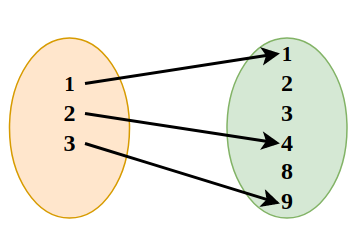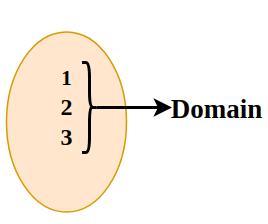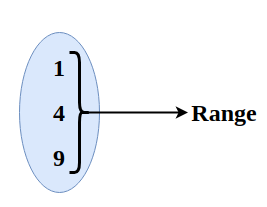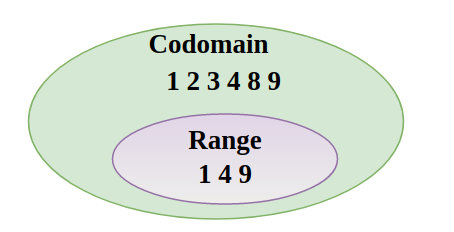Related Articles

# Introduction to Domain and Range

To understand the concept of Domain and range of a Relation first, we have to learn about Relation. A relation is a set of rules which relates the value of one set to the value of other sets.

The domain of a relation is the set of values that we take as input and the range is the set of the values which are obtained in the form of the answers to the relation.

In this article, we will learn about the Domain and Range of Relations, its examples, and others.

## What is a Relation?

For any two non-empty sets A and B, we define the relation R as the subset of the  Cartesian product of A × B where each member of set A is related to the member of set B through some unique rule.

We defined relation as,

R = {(x,y): x ∈ A and y ∈ B}

Example: The relation R = {(1,1), (2,4), (3,9)} and it is represented using {}.## Types of Relations

There are the following types of relations between two sets:

• Universal relation
• Empty relation
• Identity relation
• Symmetric relation
• Inverse relation
• Reflexive relation
• Transitive relation

## Universal Relations

A relation is called a universal connection if each element of set A is related to another element of set A i.e. R =  A × A.

## Empty Relations

The relation wherein there is no connection between any components of a set is called an empty relation. An empty relation is also called void relation.

Consider if set A = {2, 3} then, empty relation is R = {m,n} where, |m-n| = 8.

## Identity Relations

The relation where each component of a set is identified with itself is called Identity Relations.

Consider a set A = {1,2,3}, the identity relation will be I = {1,1}, {2,2}, {3,3}.

I = {(m,m), m ∈ A}

## Symmetric Relations

A relation is symmetric if a = b holds true then b = a also holds true. A connection R is symmetric just if (b, a) ∈ R is then (a,b) ∈ R.

R = {(1, 2), (2, 1)} is symmetric relation set for a set A = {1, 2}.

## Inverse Relations

Inverse relation occurs when a set has inverse pairs of another set. i.e. if R ∈ A × B then the inverse relation is R-1 = {(b,a) such that (a,b) ∈ R}

Consider if set A = {(1,2),(3,4)}, then inverse relation will be R-1 = {(2,1), (4,3)}.

## Reflexive Relations

A relation where each component of a set A is mapped to itself is called reflexive relation, i.e. for every x ∈ A, (a, a) ∈ R

Example: For set A = {-1, 4}. Reflexive relation is R = {(-1, -1), (4, 4), (-1, 4), (4, -1)}

## Transitive Relations

If for any relation (m,n) ∈ R  and (n,p) ∈ R, then if (m,p) ∈ R is

Consider aRb and bRc ⇒ aRc ∀ a, b, c ∈ A

## Domain and Range of a Relation

As we know any set of ordered pairs that are related to a unique relation we have domain and range i.e. for R such that R(A×B) such that {(a, b) where a ∈ A and b ∈ B} we have domain and range of R.

Here the set of values of A is called the domain and the set of values of b is called the range.

### Domain of a Relation

Domain of any Relation is the set of input values of the relation. For example, if we take two sets A and B, and define a relation R: {(a,b): a ∈ A, b ∈ B} then the set of values of A is called the domain of the function.

The image given below represents the domain of a relation.### Range of a Relation

Range of any Relation is the set of output values of the relation. For example, if we take two sets A and B, and define a relation R: {(a,b): a ∈ A, b ∈ B} then the set of values of B is called the domain of the function.

The image given below represents the range of a relation.### Codomain of Relation

We define the codomain of the relation R as the set B of the cartesian product A×B on which the relation is defined. Now it is clear that the range of the function is the proper subset of Codomain.

Range ⊆ CodomainExample: Take a set S = {4, 5, 6, 9,10,11,12,13, 17} and define a relation A from S to S such that in the ordered pair (x,y) in A, y is two more than x.

Solution:

We define R as,

R = {(9,11), (10,12), (11,13)}

Thus,

• Domain of R is (9, 10, 11)
• Range of R is (11, 12, 13)
• Codomain is (4, 5, 6, 9,10,11,12,13, 17)

## Solved Examples on Domain and Range of a Relation

Example 1: Find the domain and range of the relation R: {(a,a2) | a ∈ A, a2 ∈ A} which is defined on A×A and the set A = {1,2,3,4,5,6,7,8,9}.

Solution:

Relation R is defined as,

R = {(1,1), (2,4), (3,9)}

• Domain of R = {1,2,3}
• Range of R = {1,4,9}
• Codomain of R = Set A = = {1,2,3,4,5,6,7,8,9}

Example 2: Find the domain and range of the relation R: {(a, a+3) | a ∈ A, a2 ∈ A} which is defined on A×A and the set A = {1, 2, 3, 4, 5, 6, 7, 8, 9}.

Solution:

Relation R is defined as,

R = {(1,4), (2,5), (3,6), (4,7), (5,8), (6,9)}

• Domain of R = {1, 2, 3, 4, 5}
• Range of R = {4, 5, 6, 7, 8, 9}
• Codomain of R = Set A = = {1, 2, 3, 4, 5, 6, 7, 8, 9}

Example 3: Find the domain and range of the relation R: {(a, b) | a+b = 7 a ∈ A, b ∈ B} which is defined on A×B and the set A = {1, 2, 3, 4} B = {5, 6, 7, 8, 9}.

Solution:

Relation R is defined as,

R = {(1,6), (2,5)}

• Domain of R = {1, 2}
• Range of R = {6, 5}
• Codomain of R = Set B = {5, 6, 7, 8, 9}

## FAQs on Relations

### Q1: What is a Relation?

The relation is defined as the subset of the cartesian product A×B which satisfies any specific condition. It links the values of set A to the specific values of set B.

### Q2: What is the Domain of a Relation?

If a relation is defined on the cartesian product of A×B satisfying a specific condition. Then the set of values taken as input from A satisfying the relation is called the domain of the relation.

### Q3: What is the Range of a Relation?

For a relation defined on the cartesian product of A×B and satisfying a specific condition. Then the set of values as output taken from B to build the relation is called the range of the relation.

### Q4: What is a Function?

A relation in which each element of set A is uniquely mapped to the element of set B in the cartesian product of A×B is called a function.